##### Biostatistics For Dummies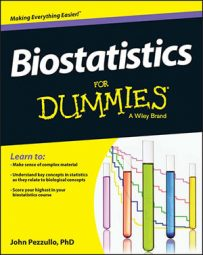To estimate sample size in biostatistics, you must state the effect size of importance, or the effect size worth knowing about. If the true effect size is less than the “important” size, you don’t care if the test comes out nonsignificant. With a few shortcuts, you can pick an important effect size and find out how many subjects you need, based on that effect size, for several common statistical tests.

All the graphs, tables, and rules of thumb here are for 80 percent power and 0.05 alpha (that is, the sample size you need in order to have an 80 percent chance of getting a p value that’s less than or equal to 0.05). If you want sample sizes for other values of power and alpha, use these simple scale-up rules:

• For 90 percent power instead of 80 percent: Increase N by a third (multiply N by 1.33).

• For α = 0.01 instead of 0.05: Increase N by a half (multiply N by 1.5).

• For 90 percent power and α = 0.01: Double N (multiply N by 2).

## Estimating sample size for correlation tests

For a correlation test in biostatistics (such as the Pearson or Spearman test), pick the scatter chart that looks like an important amount of correlation. Each chart shows the value of r (the correlation coefficient) and the required number of analyzable subjects (each providing an x and a y value). For example, if the scatter chart in the lower left corner (corresponding to r = 0.6) appears to show an important amount of correlation, you’ll need about 20 analyzable subjects.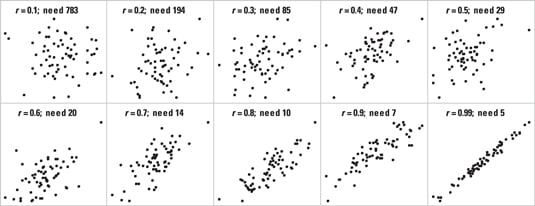Credit: Illustration by Wiley, Composition Services Graphics

For other r values that aren’t in the preceding scatter charts, use this rule of thumb to estimate sample size: You need about 8/r2 – 3 analyzable subjects.

## Sample size estimation for unpaired student t tests

In biostatistics, when comparing the means of two independent groups of subjects using an unpaired Student t test, the effect size is expressed as the ratio of Δ (delta, the difference between the means of two groups) divided by σ (sigma, the within-group standard deviation).

Each chart in the following figure shows overlapping bell curves that indicate the amount of separation between two groups, along with the effect size (Δ/σ) and the required number of analyzable subjects in each group. Pick the chart that looks like an important amount of separation between the two groups. For example, if the middle chart (corresponding to a between-group difference that’s three-fourths as large as the within-group standard deviation) looks like an important amount of separation, then you need about 29 analyzable subjects per group (for a total of 58 analyzable subjects).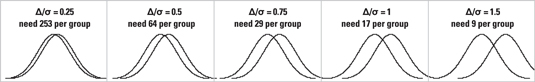Credit: Illustration by Wiley, Composition Services Graphics

For other Δ/σ values, use this rule of thumb to estimate sample size: You need about 16/(Δ/σ)2 analyzable subjects in each group.

## Sample size estimation for paired student t tests

In biostatistics, when comparing paired measurements (such as changes between two time points for the same subject) using a paired Student t test, the effect size is expressed as the ratio of Δ (delta, the mean change) divided by σ (sigma, the standard deviation of the changes). Another, perhaps easier, way to express the effect size is by the relative number of expected subjects with positive versus negative changes. (These ratios are shown below each curve.)

Each chart in the following figure shows a bell curve indicating the spread of changes, along with the effect size (Δ/σ), the ratio of positive to negative differences, and the required number of analyzable subjects (each subject providing a pair of measurements). Pick the chart that looks like an important amount of change (relative to the vertical line representing no change). For example, the middle chart corresponds to a mean change that is three-fourths as large as the standard deviation of the changes, with about 3.4 times as many subjects increasing as decreasing. If this looks like an important amount of change, then you need 16 pairs of measurements (such as 16 subjects, each with a pre-treatment and a post-treatment value).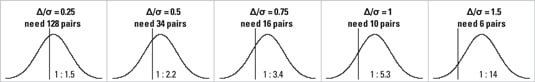Credit: Illustration by Wiley, Composition Services Graphics

For other Δ/σ values, use this rule of thumb to estimate sample size: You need about 8/(Δ/σ)2 + 2 pairs of measurements.

## Estimating sample size when comparing two proportions

The proportion of subjects having some attribute (such as responding to treatment) can be compared between two groups of subjects by creating a cross-tab from the data, where the two rows represent the two groups, and the two columns represent the presence or absence of the attribute. In biostatistics, this cross-tab can be analyzed with a chi-square or Fisher Exact test.

To estimate the required sample size, you need to provide the expected proportions in the two groups. Look up the two proportions you want to compare at the left and top of the following table. (It doesn’t matter which proportion you look up on which side.) The number in the cell of the table is the number of analyzable subjects you need in each group. (The total required sample size is twice this number.)

For example, if you expect 40 percent of untreated subjects with a certain disease to die but only 30 percent of subjects treated with a new drug to die, you would find the cell at the intersection of the 0.30 row and the 0.40 column (or vice versa), which contains the number 376. So you need 376 analyzable subjects in each group, or 752 analyzable subjects altogether.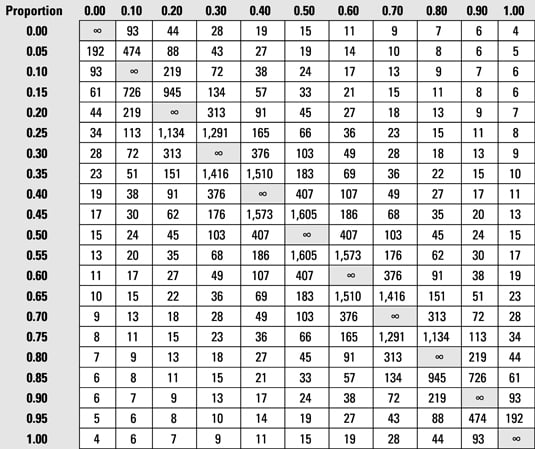Credit: Illustration by Wiley, Composition Services Graphics